Reconstruction of the 2021 Kitt's Lane formation 1.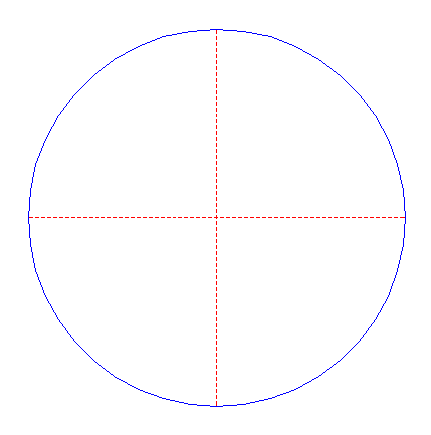Draw a circle. Draw the horizontal and vertical centerlines. 2.Construct two inscribed squares of circle 1, one pointing up, one with horizontal and vertical sides. 3.Construct eight inscribed equilateral triangles of circle 1, each with one angular point coincident with consecutive angular points of squares 2. 4.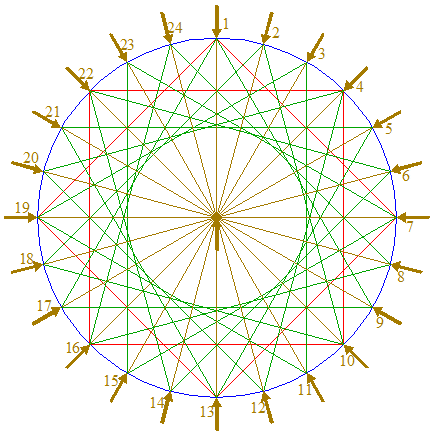Draw twentyfour rays of circle 1, from the center to the angular points of triangles 3. Number these rays clockwise 1 - 24, starting at the top. 5.Construct the inscribed circle of squares 2. 6.Construct a circle concentric to circle 1, passing through the intersection of ray 4 nr. 2 and the upper horizontal side of squares 2. 7.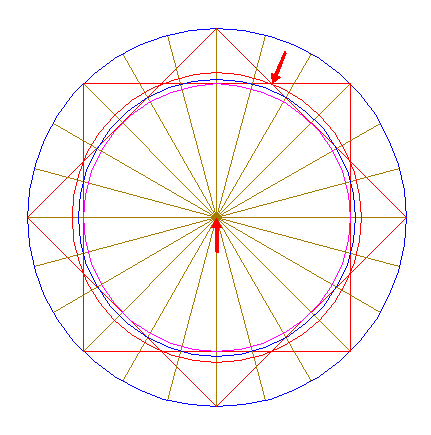Construct a circle concentric to circle 1, passing through the intersections of squares 2. 8.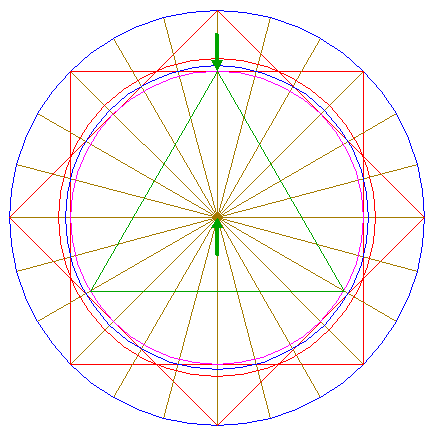Construct the inscribed equilateral triangle of circle 5, pointing up. 9.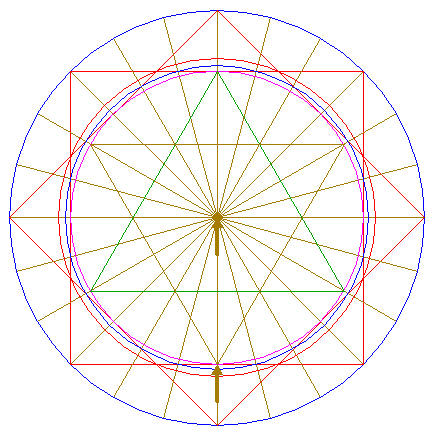Construct the inscribed equilateral triangle of circle 5, pointing down. 10.Construct a circle centered at the upper intersection of squares 2 to the left, passing through the corresponding intersection to the right. 11.Copy circle 10 to the center of circle 1. 12.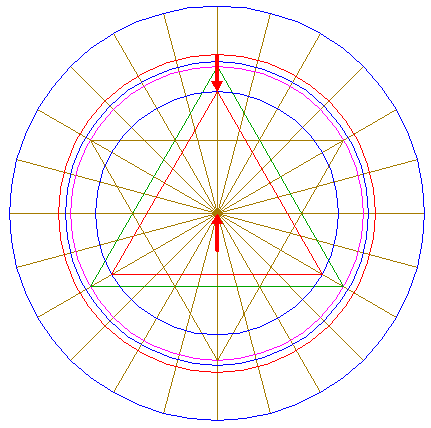Construct the inscribed equilateral triangle of circle 11, pointing up. 13.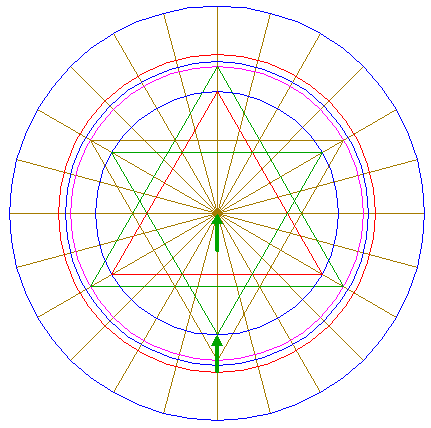Construct the inscribed equilateral triangle of circle 11, pointing down. 14.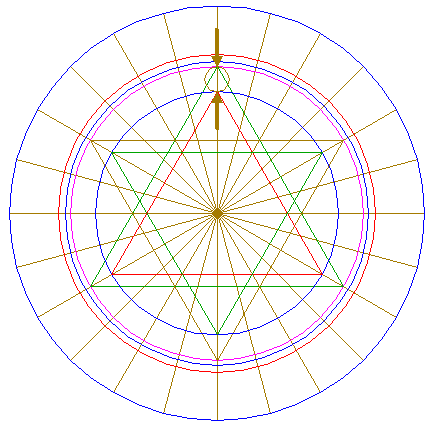Construct the "two-points" circle (defined by the two end-points of a centerline) between the upper intersections of circles 5 and 11 with the vertical centerline. 15.Construct a circle concentric to circle 1, passing through the center of circle 14. 16.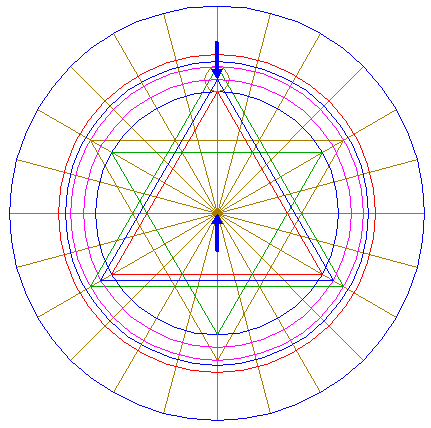Construct the inscribed equilateral triangle of circle 15, pointing up. 17.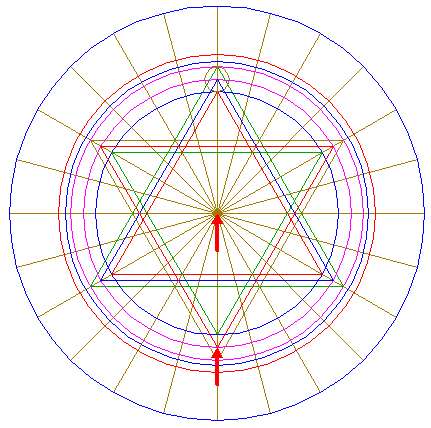Construct the inscribed equilateral triangle of circle 15, pointing down. 18.Copy circle 14 to the top of triangle 12. 19.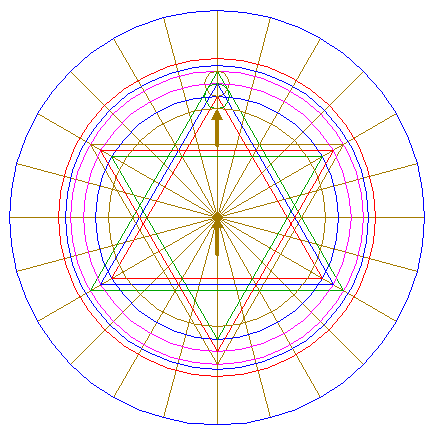Construct a circle concentric to circle 1, tangent to circle 18 at the lower side. 20.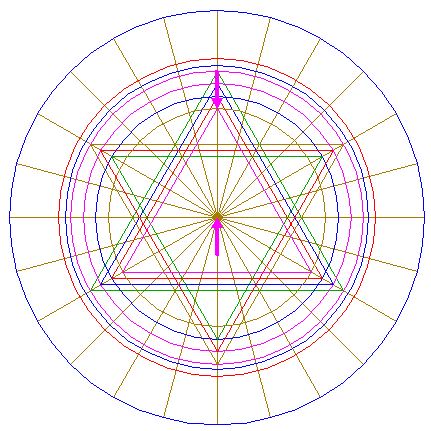Construct the inscribed equilateral triangle of circle 19, pointing up. 21.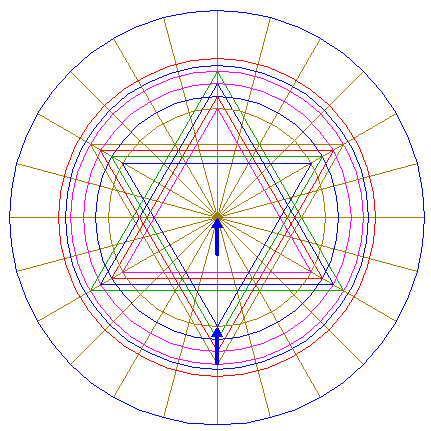Construct the inscribed equilateral triangle of circle 19, pointing down. 22.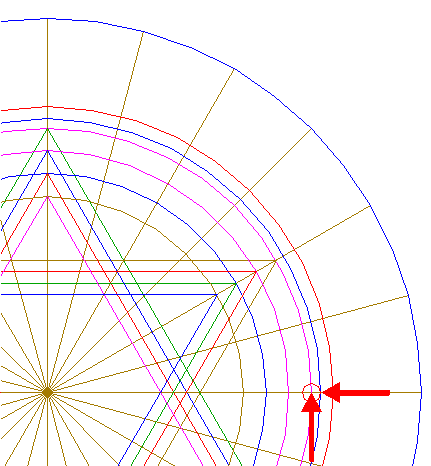Construct a circle centered at the righthand intersection of circle 5 and the horizontal centerline, tangent to circle 6 at the righthand side. 23.Copy circle 22 to the upper intersection of circle 1 and the vertical centerline. 24.Construct a circle concentric to circle 1, tangent to circle 23 at the lower side. 25.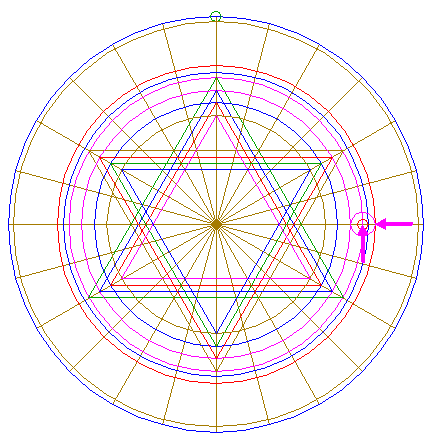Construct a circle concentric to circle 22, tangent to circle 7 at the righthand side. 26.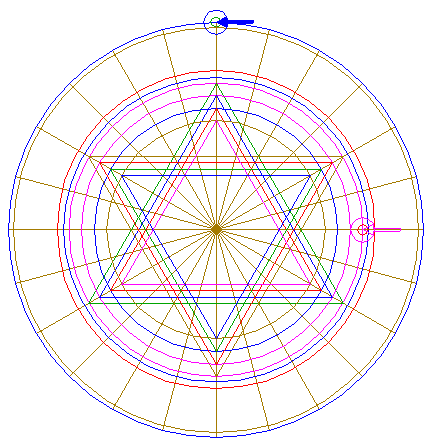Copy circle 25 to the center of circle 23. 27.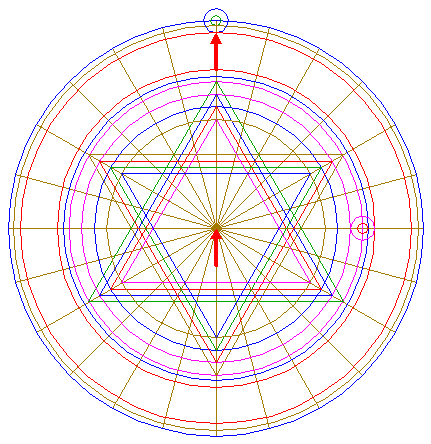Construct a circle concentric to circle 1, tangent to circle 26 at the lower side. 28.Construct three circles centered at the intersection of the lower side of triangle 20 and the vertical centerline, tangent to the lower sides of triangles 8, 12, and 16. 29.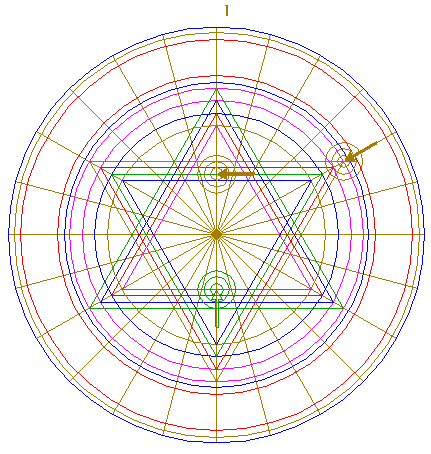Copy circles 28 two times, to the intersection of triangle 13 and ray 4 nr. 1, and to the upper righthand angular point of triangle 9. 30.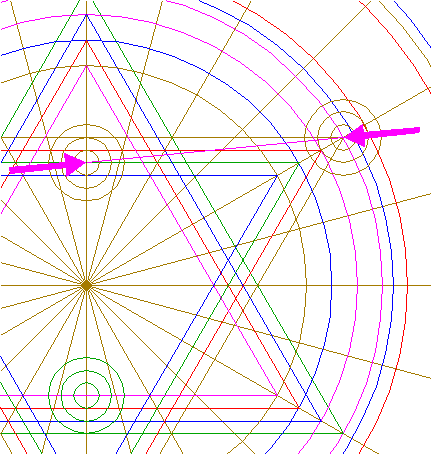Draw the connecting line between the centers of circles 29. 31.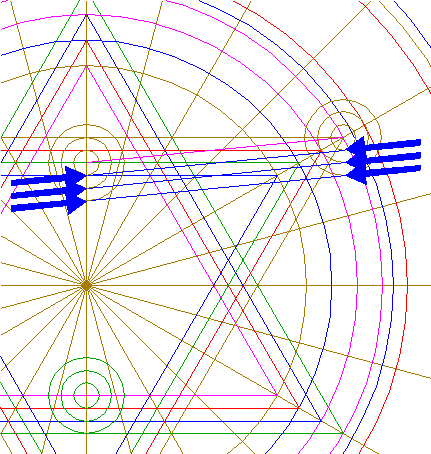Draw three parallel lines, parallel to line 30, tangent to circles 29 at the lower sides. 32.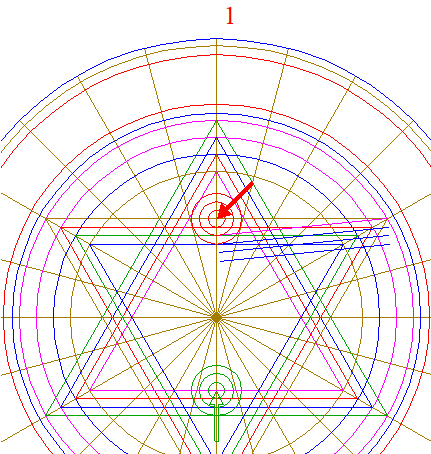Copy circles 28 to the intersection of triangle 9 and ray 4 nr. 1. 33.Draw three parallel lines, parallel to the vertical centerline, tangent to circles 28 and 32 at the righthand sides. 34.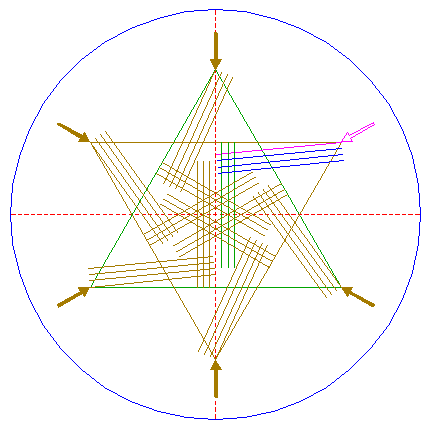Repeat all necessary steps to copy and rotate lines 30, 31, and 33 five times, with respect to the other angular points of triangles 8 and 9, as shown. 35.Remove all redundant parts from rays 4, triangles 8, 9, 12, 13, 16, 17, 20, and 21, and lines 30, 31, 33, and 34, such that... 36.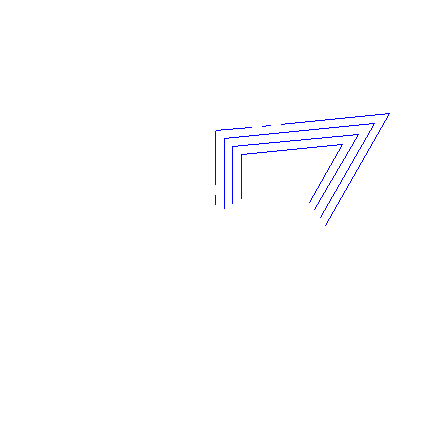...this pattern remains. 37.Repeat all necessary steps to copy and rotate pattern 36 five times, with respect to the other angular points of triangles 8 and 9, as shown. 38.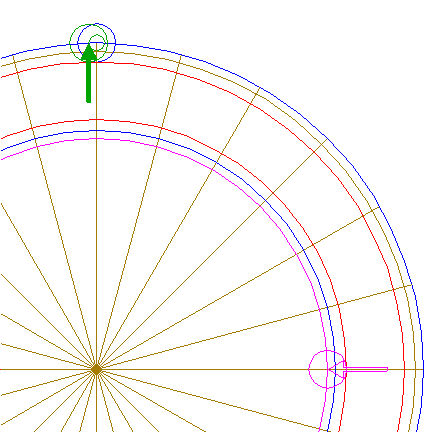Copy circle 25 to the lefthand intersection of circles 1 and 23. 39.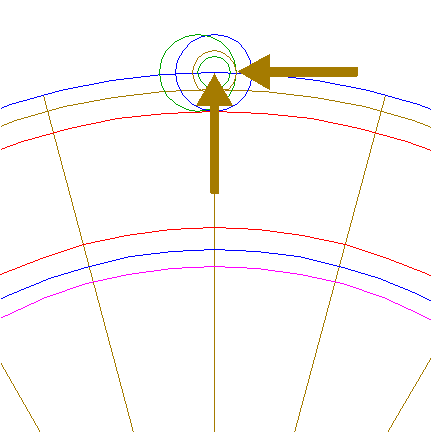Construct a circle concentric to circle 23, tangent to circle 38 at the righthand side. 40.Copy circles 23, 26, and 39 five times, to the intersections of circle 1 with rays 4 nrs. 2 and 24, and to the intersections of circle 5 with rays 4 nrs. 1, 2, and 24. 41.Draw three parallel lines, parallel to ray 4 nr. 1, tangent to circles 23, and 26, at the lefthand sides, and circle 39 at the righthand side, and tangent to the corresponding circles 40 at the corresponding sides, as shown. 42.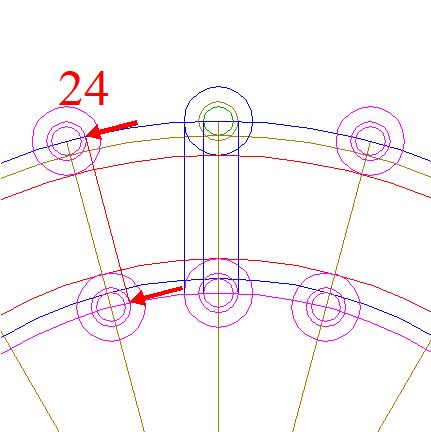Draw a line parallel to ray 4 nr. 24, tangent to the relevant middle circles 40, at the righthand sides, as shown. 43.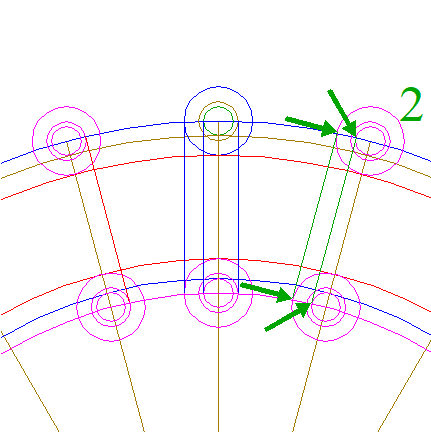Draw two parallel lines, parallel to ray 4 nr. 2, tangent to the relevant outer and inner circles 40, both at the lefthand sides, as shown. 44.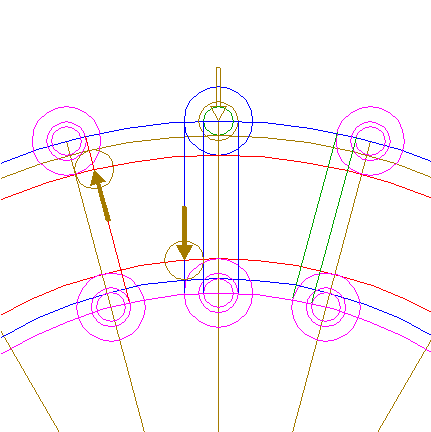Copy circle 39 two times, one to the intersection of circle 7 and the leftmost line 41, one to the intersection of circle 27 and line 42. 45.Copy circle 39 two times, one to the intersection of circle 7 and the rightmost line 41, one to the intersection of circle 27 and the lefthand line 43. 46.Draw the connecting line between the centers of circles 44. 47.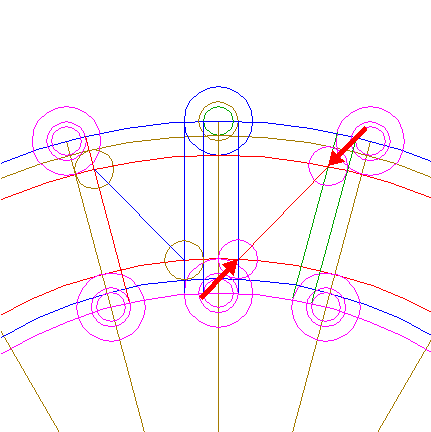Draw the connecting line between the centers of circles 45. 48.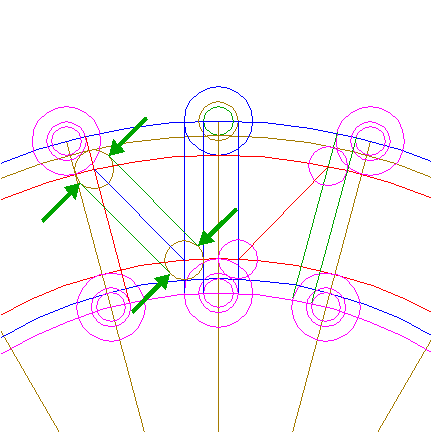Draw two parallel lines, parallel to line 46, tangent to circles 44 at both sides. 49.Draw two parallel lines, parallel to line 47, tangent to circles 45 at both sides. 50.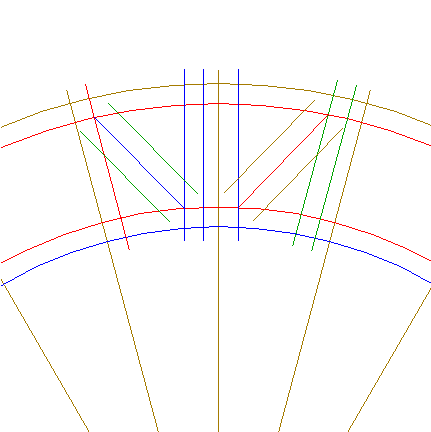Remove all redundant parts from rays 4, circles 6, 7, 24, and 27, and lines 41, 42, 43, 46, 47, 48, and 49, such that the shown patterns remain.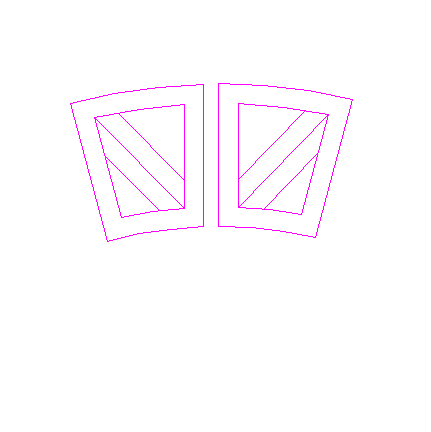51.Repeat all necessary steps to copy and rotate patterns 50 eleven times, with respect to rays 4 nrs. 3, 5, 7, 9, 11, 13, 15, 17, 19, 21, and 23. 52.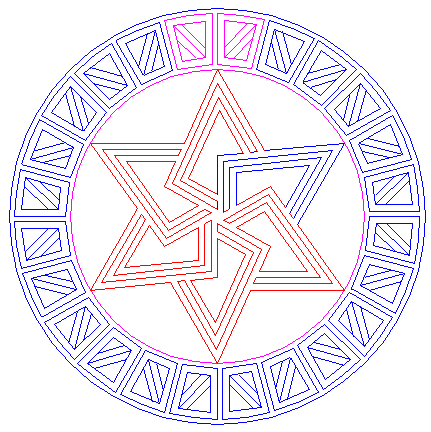Circles 1, and 5, and patterns 36, 37, 50, and 51, together, make up the final reconstruction. 53.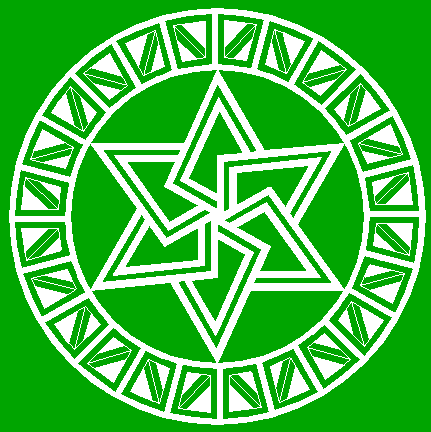Colour all areas corresponding to standing... 54....or to flattened crop, and finish the reconstruction of the 2021 Kitt's Lane formation. 55.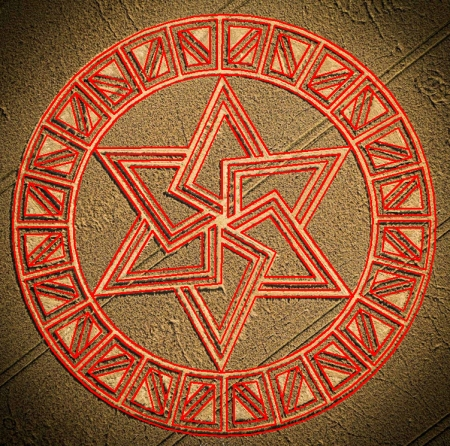The final result, matched with two aerial images.